Next: Fourier analysis and reconstruction Up: Fourier analysis and resynthesis Previous: Fourier transform of a   Contents   Index

# Fourier analysis of non-periodic signals

Most signals aren't periodic, and even a periodic one might have an unknown period. So we should be prepared to do Fourier analysis on signals without making the comforting assumption that the signal to analyze repeats at a fixed period. Of course, we can simply takesamples of the signal and make it periodic; this is essentially what we did in the previous section, in which a pure sinusoid gave us the complicated Fourier transform of Figure 9.3 (part b).

However, it would be better to get a result in which the response to a pure sinusoid were better localized around the corresponding value of. We can accomplish this using the enveloping technique first introduced in Figure 2.7 (Page). Applying this technique to Fourier analysis will not only improve our analyses, but will also shed new light on the enveloping looping sampler of Chapter 2.

Given a signal, periodic or not, defined on the points fromto, the technique is to envelope the signal before doing the Fourier analysis. The envelope shape is known as a window function. Given a window function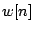, the windowed Fourier transform is:Much ink has been spilled over the design of suitable window functions for particular situations, but here we will consider the simplest one, named the Hann window function (the name is sometimes corrupted to Hanning" in DSP circles). The Hann window is:It is easy to analyze the effect of multiplying a signal by the Hann window before taking the Fourier transform, because the Hann window can be written as a sum of three complex exponentials:where as before,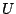is the unit-magnitude complex number with argument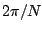. We can now calculate the windowed Fourier transform of a sinusoidwith angular frequencyas before. The phases come out messy and we'll replace them with simplified approximations: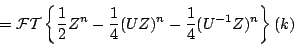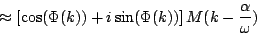where the (approximate) phase term is:and the magnitude function is: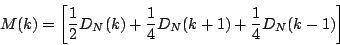The magnitude function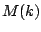is graphed in Figure 9.5. The three Dirichlet kernel components are also shown separately.The main lobe ofis four harmonics wide, twice the width of the main lobe of the Dirichlet kernel. The sidelobes, on the other hand, have much smaller magnitude. Each sidelobe ofis a sum of three sidelobes of, one attenuated byand the others, opposite in sign, attenuated by. They do not cancel out perfectly but they do cancel out fairly well.

The sidelobes reach their maximum amplitudes near their midpoints, and we can estimate their amplitudes there, using the approximation:Settinggives sidelobe amplitudes, relative to the peak height, of:The sidelobes drop off progressively more slowly so that the tenth one is only attenuated about 30 dB and the 32nd one about -40 dB. On the other hand, the Hann window sidelobes are attenuated by:and,,, anddB for the next four sidelobes.

This shows that applying a Hann window before taking the Fourier transform will better allow us to isolate sinusoidal components. If a signal has many sinusoidal components, the sidelobes engendered by each one will interfere with the main lobe of all the others. Reducing the amplitude of the sidelobes reduces this interference.Figure 9.6 shows a Hann-windowed Fourier analysis of a signal with two sinusoidal components. The two are separated by about 5 times the fundamental frequency, and for each we see clearly the shape of the Hann window's Fourier transform. Four points of the Fourier analysis lie within the main lobe ofcorresponding to each sinusoid. The amplitude and phase of the individual sinusoids are reflected in those of the (four-point-wide) peaks. The four points within a peak which happen to fall at integer valuesare successively about one half cycle out of phase.

To fully resolve the partials of a signal, we should choose an analysis sizelarge enough so thatis no more than a quarter of the frequency separation between neighboring partials. For a periodic signal, for example, the partials are separated by the fundamental frequency. For the analysis to fully resolve the partials, the analysis periodmust be at least four periods of the signal.

In some applications it works to allow the peaks to overlap as long as the center of each peak is isolated from all the other peaks; in this case the four-period rule may be relaxed to three or even slightly less.Next: Fourier analysis and reconstruction Up: Fourier analysis and resynthesis Previous: Fourier transform of a   Contents   Index
Miller Puckette 2006-12-30